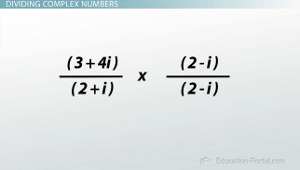# How to Divide Complex Numbers

An error occurred trying to load this video.

Try refreshing the page, or contact customer support.

Coming up next: How to Graph a Complex Number on the Complex Plane

### You're on a roll. Keep up the good work!

Replay
Your next lesson will play in 10 seconds
• 0:06 Complex Numbers
• 0:39 Complex Conjugates
• 1:19 Multiplying Conjugates
• 2:08 Dividing Complex Numbers
• 6:03 Lesson Summary

Want to watch this again later?

Timeline
Autoplay
Autoplay
Speed

#### Recommended Lessons and Courses for You

Lesson Transcript
Instructor: Luke Winspur

Luke has taught high school algebra and geometry, college calculus, and has a master's degree in education.

While adding, subtracting and multiplying complex numbers is pretty straightforward, dividing them can be pretty tricky. It comes down to the process of multiplying by the complex conjugate. Learn about what that is, and how to do it, here.

## Complex Numbers

Complex numbers are a combination of a real number with an imaginary one. Because of that, we can express them generally as a + bi, where a is the real part of the number and b is the imaginary part. As long as you remember that i^2 = -1, then adding, subtracting and multiplying them is really just a review of combining like terms and multiplying binomials with FOIL. But when it comes to dividing complex numbers, some new skills are going to need to be learned.

## Complex ConjugatesAlong with these new skills, you're going to need to remind yourself what a complex conjugate is. If you haven't heard of this before, don't worry; it's pretty straightforward. A conjugate is a binomial where the sign on the second term has been switched. So the complex conjugate to a + bi is just a - bi, and visa versa. Here are some examples of complex conjugates: 2 + 3i and 2 - 3i, or -3 - i and -3 + i or 4 - 5i and 4 + 5i. Just remember that the sign on the first term stays the same, but the sign on the second one switches.

## Multiplying Conjugates

Conjugates are special because there is a pattern to multiplying them. Let's show this with two of our examples from above: 2 + 3i and 2 - 3i. Multiplying these two complex numbers with FOIL will give us 4 - 6i + 6i - 9i^2. But then when we combine like terms, the two groups of i's in the middle are going to cancel out. Substituting in -1 for i^2, and then doing 4 + 9 gives us our answer as 13, a real number!

Multiplying any two conjugates will make these middle terms cancel out. Which means that multiplying two complex conjugates will always make the i's cancel out, leaving us with a real number every time: (a + bi)(a - bi) = a^2 + b^2. Okay, so why do I bring this up? Well, dividing complex numbers will take advantage of this trick.

## Dividing Complex NumbersThere really isn't a good way to figure out how many of these complex numbers fit into that one, so instead we do some fancy hand waving (using the conjugate) to make the imaginary part of the complex number we're dividing by go away. Then it's just dividing by a real number, something that we know how to do.

Whew, that's a lot of words; let me show you what I mean. Take the example (3 + 4i) / (2 + i). Like I said earlier, I have no idea how many 2 + is fit into 3 + 4i, so writing the problem this way doesn't really give us much. Instead, changing it into a fraction will allow us to begin using our fancy math tricks. If we now remember what we just learned about the complex conjugate, we can change this from dividing by a complex number (something we don't know how to do) into dividing by a real number (something we do know how to do). So we want to multiply the denominator by its conjugate, 2 - i (that would make it a real number), but we can't just do anything we want. We have to leave the problem the same. Multiplying by some random number will change it, but multiplying by 1 is actually not going to change it at all.

To unlock this lesson you must be a Study.com Member.

### Register to view this lesson

Are you a student or a teacher?

### Unlock Your Education

#### See for yourself why 30 million people use Study.com

##### Become a Study.com member and start learning now.
Back
What teachers are saying about Study.com

### Earning College Credit

Did you know… We have over 160 college courses that prepare you to earn credit by exam that is accepted by over 1,500 colleges and universities. You can test out of the first two years of college and save thousands off your degree. Anyone can earn credit-by-exam regardless of age or education level.